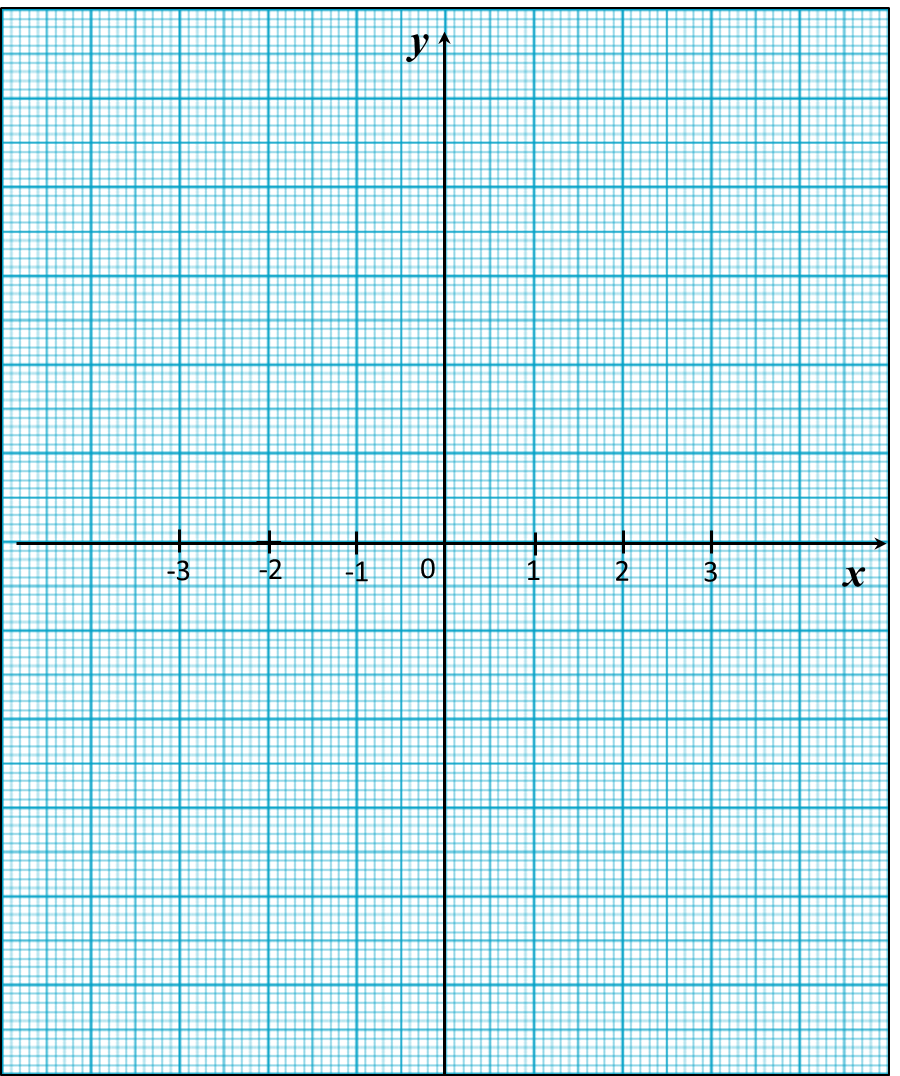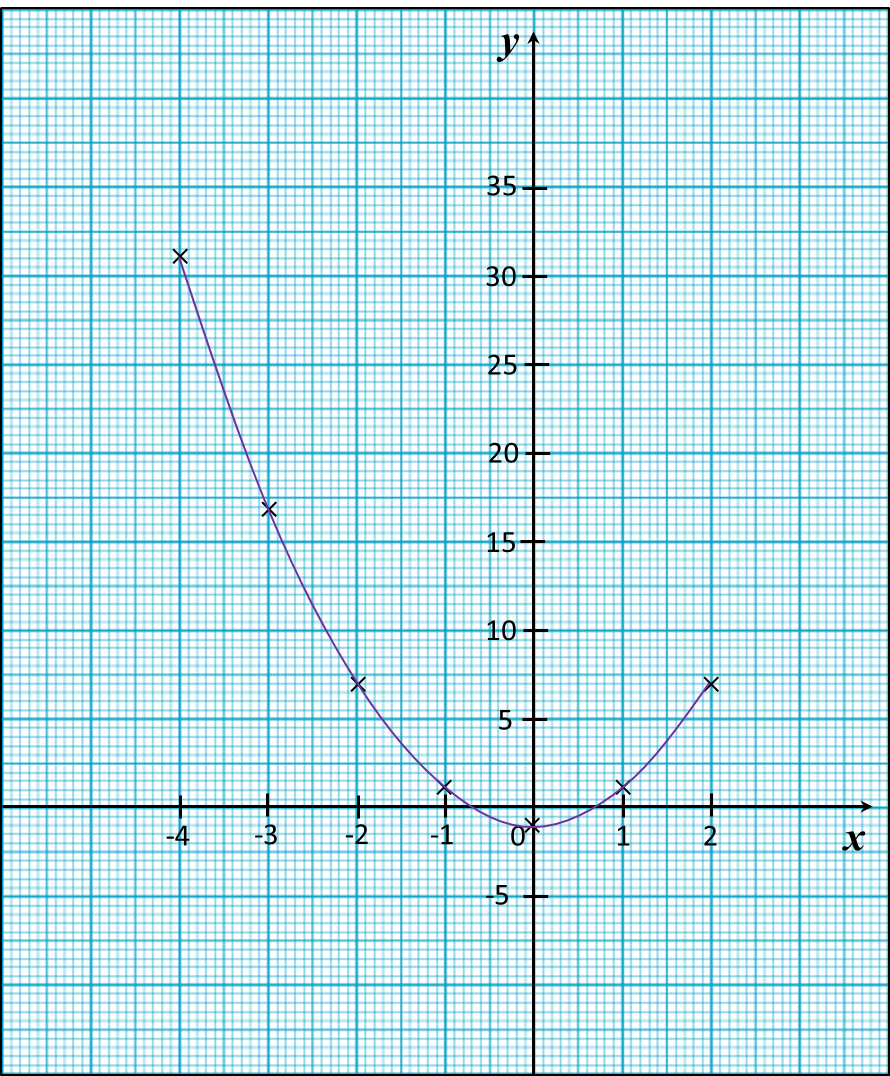13.2.2 Graphs of Functions, PT3 Focus Practice

13.2.2 Graphs of Functions, PT3 Focus Practice

Question 4:
Use graph paper to answer this question.
Table below shows the values of two variables, x and y, of a function.

 x –3 –2 –1 0 1 2 3 y –19 –3 1 –1 –3 1 17
The x-axis and the y-axis are provided on the graph paper on the answer space.
(a) By using a scale of 2 cm to 5 units, complete and label the y-axis.
(b) Based on the table above, plot the points on the graph paper.
(c) Hence, draw the graph of the function.Solution:Question 5:
Use graph paper to answer this question.
Table below shows the values of two variables, x and y, of a function.

 x –4 –3 –2 –1 0 1 2 y 31 17 7 1 –1 1 7
The x-axis and the y-axis are provided on the graph paper on the answer space.
(a) By using a scale of 2 cm to 5 units, complete and label the y-axis.
(b)   Based on the table above, plot the points on the graph paper.
(c) Hence, draw the graph of the function.Solution:Question 6:
(a) Complete table below in the answer space for the equation L = x2 + 5x by writing the value of L when x = 2.
(b) Use graph paper to answer this part of the question. You may use a flexible curve rule.
By using a scale of 2 cm to 1 unit on the x-axis and 2 cm to 5 units on the y-axis, draw the graph of L = x2 + 5x for 0 ≤ x ≤ 4.